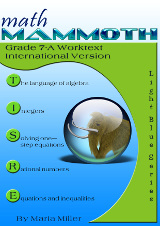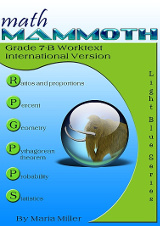^
You are here: HomeMM International Version → Grade 1

# Math Mammoth Grade 7 International Version

Math Mammoth Grade 7 International Version is a complete maths program for seventh grade. It consists of two student worktexts (A and B), a separate answer key for each, tests, mixed revisions, and in the download version, an additional worksheet maker (Internet access required) to make extra practice worksheets when needed.228 pages
202 lesson pages288 pages
253 lesson pages

## Prices & ordering

(part A and B worktexts, answer keys,
tests, mixed revisions, worksheet
maker, and Soft-Pak)

Part 7-A only: \$19.75
(part A worktext, part A answer key,
tests, mixed revisions, worksheet
maker, and Soft-Pak)

Part 1-B only: \$19.75
(part B worktext, part B answer key,
tests, mixed revisions, worksheet
maker, and Soft-Pak)

#### Printed copies

You can purchase printed hardcopies at Lulu. The prices below are in USD.

7-A worktext \$16.95
7-B worktext \$19.75
Tests & Mixed Revisions \$11.95

This curriculum is essentially the same as the US version of Math Mammoth Grade 7. The program is customised for the international audience in these aspects:

• The curriculum teaches the metric measurement units. Imperial units, such as inches and pounds, are not used.
• The spelling conforms to British international standards (British English).
• Page (paper) size is A4.
• Large numbers are formatted with a space as the thousands separator (such as 12 394). (The decimals are formatted with a decimal point, like in the US version.)

In money-related word problems, the symbol it uses is "\$" (dollar).

## Introduction

In the book 7-A, we start out with an introduction to basic algebra, which is in many ways a revision of the same topics from 6th grade. The first chapter revises the order of operations, the concepts of an expression and equation, and the distributive property. Students learn about the commutative and associative properties of addition and multiplication, and they simplify expressions that do not involve negative numbers.

In chapter 2, we study integers and their operations in detail. Some of this is revision from 6th grade, and some of it is new. The four operations of integers are explained with the help of two visual models: the number line and counters, hopefully providing an intuitive understanding of the processes. Students need to be able to add, subtract, multiply, and divide integers when they learn to solve equations in chapter 3. In the end of the chapter students also learn about negative fractions.

In the next chapter (chapter 3) students study simple one-step equations. They already know the basics of how to solve this type of equations from 6th grade, but this time we use negative numbers in them.

Chapter 4 is titled Rational Numbers, which are simply fractions and certain decimals, so the student is already very familiar with them. The goal of the chapter is to be able to add, subtract, multiply, and divide both positive and negative fractions and decimals. We also solve simple equations involving fractions and decimals and learn about scientific notation.

The last chapter in part 7-A focuses on linear equations. The student learns to solve various types of linear equations and practises using those in simple word problems. We study linear inequalities but not to the same depth as linear equations. Lastly, the student graphs linear equations and is introduced to the concept of slope, which is the steepness of a line. The student can continue studying these topics with more details in an Algebra 1 course.

Part B starts out with a study of ratios and proportions (chapter 6). Students study unit rates, proportions, proportional relationships and graphing, scaling geometric figures, floor plans, and maps.

The next chapter provides thorough lessons on the concept of percent. Students learn to solve a wide variety of problems involving percentages, including percentage of change, percentages of comparison, and simple interest problems.

Geometry is our focus in chapter 8. Students draw geometric figures using a a protractor and a ruler, and they also learn some basic geometric constructions. The other themes of this chapter are various angle relationships, area and the perimeter of a circle, conversions between units of area and of volume, surface area, volume, and cross- sections when solids are sliced with a plane.

Chapter 9 covers square roots, the Pythagorean Theorem, and its applications. I have included the Pythagorean Theorem in order to make the curriculum work as a pre-algebra course, and you can omit the entire chapter 9 if you are following the Common Core Standards for grade 7.

Chapter 10 is an introduction to probability. Besides learning the basic idea behind probability as the ratio of favourable events to all possible events, students compare experimental probabilities to theoretical ones in probability simulations and even design some on their own.

Lastly, in chapter 11, the curriculum covers statistical concepts. The major areas of study are random sampling and learning to compare two populations using some basic statistical measures and graphs.

Please also see the table of contents for 7-A and 7-B (in the sample), which will let you see the topics covered in more detail.

Some main features of Math Mammoth complete curriculum (Light Blue series) are:

• focuses on understanding of mathematical concepts
• uses clear explanations, lots of visual exercises and pattern exercises
• mastery oriented: concentrates at length on a topic, with very few topics per grade
• emphasizes mental maths and developing number sense
• very little teacher preparation needed

The downloadable version includes a worksheet maker (Internet access required) that lets you make extra practice worksheets for many of the topics in the curriculum.

The PDFs in the download version are enabled for annotation. This means that if you prefer, your student can fill them in on the computer, using the typewriter and drawing tools in Acrobat Reader version 9 or greater.

## How and where to order

You can buy Math Mammoth International Version:

• As a digital version on this site (Mathmammoth.com) — using the "Add to cart" buttons.
• As a print version at Lulu. Follow the links from this site to Lulu.

Also available:

Math Mammoth Grades 1-3 Bundle, International Version, \$79 USD

Get grades 1-3 in a discounted bundle — 33% off! This means you save \$39.50 USD (or the equivalent in your currency).

Math Mammoth Grades 4-7 Bundle, International Version, \$105 USD

Get grades 4-7 in a discounted bundle — 33% off! This means you save \$53 USD (or the equivalent in your currency).

By purchasing any of the books, permission IS granted for the purchaser (teacher or parent) to reproduce this material to be used with his/her students in a teaching situation; not for commercial resale. Please note: you are not permitted to share the material with other teachers or parents.

In other words, you are permitted to make copies for the students/children you are teaching, but not for other teachers' usage.

WAIT!

Receive my monthly collection of math tips & resources directly in your inbox — and get a FREE Math Mammoth book!You can unsubscribe at any time.

### Math Mammoth Tour

Confused about the different options? Take a virtual email tour around Math Mammoth! You'll receive:

An initial email to download your GIFT of over 400 free worksheets and sample pages from my books. Six other "TOURSTOP" emails that explain the important things and commonly asked questions concerning Math Mammoth curriculum. (Find out the differences between all these different-colored series!)

This way, you'll have time to digest the information over one or two weeks, plus an opportunity to ask me personally about the curriculum.
A monthly collection of math teaching tips & Math Mammoth updates (unsubscribe any time)### "Mini" Math Teaching Course

This is a little "virtual" 2-week course, where you will receive emails on important topics on teaching math, including:

- How to help a student who is behind
- Troubles with word problems
- Teaching multiplication tables
- Why fractions are so difficult
- The value of mistakes
- Should you use timed tests
- And more!

A GIFT of over 400 free worksheets and sample pages from my books right in the very beginning.A monthly collection of math teaching tips & Math Mammoth updates (unsubscribe any time)
Enter your email to receive math teaching tips, resources, Math Mammoth news & sales, humor, and more! I tend to send out these tips about once monthly, near the beginning of the month, but occasionally you may hear from me twice per month (and sometimes less often).• A GIFT of over 400 free worksheets and sample pages from my books.